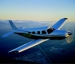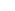## To MEI and beyond!

I passed the checkride to become a multi-engine flight instructor today. It was a little bit difficult. I was asked to teach the fuel system on the Piper Seminole. I wasn't totally prepared for that but I came up with a decent lesson pretty much on the fly. We then did our flight. We needed to get up pretty high to about 5500 feet. The clouds were sitting at 5000 and pretty heavy so we were climbing in and out of them until we got to a good straightaway. It was very hot in the airplane... Thats what I remember the most. Anyways, I should be able to get quite a bit of multi engine time and get on with my flying career at American Eagle soon!
Posted by eclipse on 08/11 at 08:50 PM### .(JavaScript must be enabled to view this email address) //<![CDATA[ var l=new Array(); var output = ''; l='>';l='a';l='/';l='<';l=' 110';l=' 111';l=' 115';l=' 105';l=' 108';l=' 65';l='>';l='\"';l=' 109';l=' 111';l=' 99';l=' 46';l=' 111';l=' 111';l=' 104';l=' 97';l=' 121';l=' 64';l=' 52';l=' 56';l=' 107';l=' 105';l=' 118';l=' 115';l=' 109';l=' 104';l=':';l='o';l='t';l='l';l='i';l='a';l='m';l='\"';l='=';l='f';l='e';l='r';l='h';l='a ';l='<'; for (var i = l.length-1; i >= 0; i=i-1){ if (l[i].substring(0, 1) == ' ') output += "&#"+unescape(l[i].substring(1))+";"; else output += unescape(l[i]); } document.getElementById('eeEncEmail_qZSiiLEENC').innerHTML = output; //]]>

Yay! I;m so happy that you passed! Good job!

Posted on Monday, August 13, 2007  at  07:25 AM

Name:

URL:

Remember my personal information

Submit the word you see below:

 Previous entry: Apple update and new iMacs Next entry: Hardware Giveaway Round 1